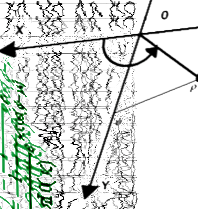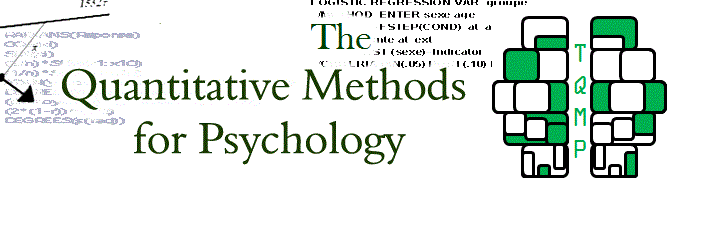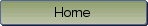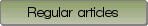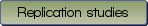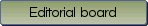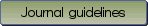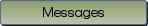Understanding statistical power using noncentral probability distributions: Chi-squared, G-squared, and ANOVA

Bibliographic information: BibTEX format RIS format XML format APA style
Cited references information: BibTEX format APA style

Hélie, Sébastien
63-69
Keywords: Statistics , statistical power , chi-square and anova
Tools: Mathematica
(no sample data)   (no appendix)

This paper presents a graphical way of interpreting effect sizes when more than two groups are involved in a statistical analysis. This method uses noncentral distributions to specify the alternative hypothesis, and the statistical power can thus be directly computed. This principle is illustrated using the chi-squared distribution and the F distribution. Examples of chi-squared and ANOVA statistical tests are provided to further illustrate the point. It is concluded that power analyses are an essential part of statistical analysis, and that using noncentral distributions provides an argument in favour of using a factorial ANOVA over multiple t tests.

Be informed of the upcoming issues with RSS feed:RSS